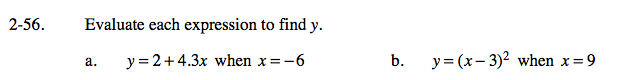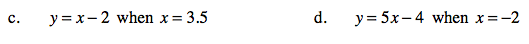### Home > CAAC > Chapter 2 > Lesson 2.1.5 > Problem2-56

2-56.Substitute −6 in for x.
y = 2 + 4.3( −6 )

Multiply and simplify.

y = 2 + (−25.8)

y = −23.8

Follow the steps in part (a).

Don't forget to use the correct order of operations.Follow the steps in part (a).

Follow the steps in part (a).

−14